# NCERT Solutions for Class 10 Maths Exercise 7.3## myCBSEguide App

CBSE, NCERT, JEE Main, NEET-UG, NDA, Exam Papers, Question Bank, NCERT Solutions, Exemplars, Revision Notes, Free Videos, MCQ Tests & more.

NCERT solutions for Maths Coordinate Geometry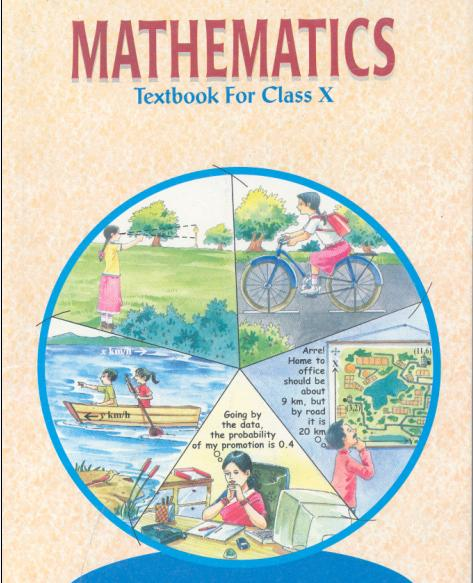## NCERT Solutions for Class 10 Maths Coordinate Geometry

###### 1. Find the area of the triangle whose vertices are:

(i) (2, 3), (–1, 0), (2, –4)

(ii) (–5, –1), (3, –5), (5, 2)

Ans. (i) (2, 3), (–1, 0), (2, –4)

Area of Triangle =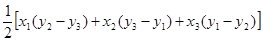=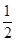[2 {0 − (−4)} – 1 (−4 − 3) + 2 (3 − 0)]

=[2 (0 + 4) – 1 (−7) + 2 (3)]

=(8 + 7 + 6) =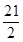sq. units

(ii) (–5, –1), (3, –5), (5, 2)

Area of Triangle ==[−5 (−5 − 2) + 3 {2 − (−1)} + 5 {−1 − (−5)}]

=[−5 (−7) + 3 (3) + 5 (4)]

=(35 + 9 + 20)

=(64) = 32 sq. units

NCERT Solutions for Class 10 Maths Exercise 7.3

###### 2. In each of the following find the value of ‘k’, for which the points are collinear.

(i) (7, –2), (5, 1), (3, k)

(ii) (8, 1), (k, –4), (2, –5)

Ans. (i) (7, –2), (5, 1), (3, k)

Since, the given points are collinear, it means the area of triangle formed by them is equal to zero.

Area of Triangle =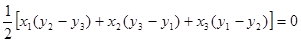[7 (1 − k) + 5 {k − (−2)} + 3 (−2 − 1)]

=(7 − 7k + 5k + 10 − 9) = 0(7 − 7k + 5k + 1) = 0(8 − 2k) = 0

⇒ 8 − 2k = 0

⇒ 2k = 8

⇒ k = 4

(ii) (8, 1), (k, –4), (2, –5)

Since, the given points are collinear, it means the area of triangle formed by them is equal to zero.

Area of Triangle =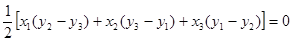[8 {−4 − (−5)} + k (−5 − 1) + 2 {1 − (−4)}]

=(8 − 6k + 10) = 0(18 − 6k) = 0

⇒18 − 6k = 0

⇒ 18 = 6k

⇒ k = 3

NCERT Solutions for Class 10 Maths Exercise 7.3

###### 3. Find the area of the triangle formed by joining the mid–points of the sides of the triangle whose vertices are (0, –1), (2, 1) and (0, 3). Find the ratio of this area to the area of the given triangle.

Ans. Let A = (0, –1) =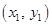, B = (2, 1) =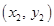and

C = (0, 3) =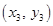Area of △ABC =⇒ Area of △ABC

=[0 (1 − 3) + 2 {3 − (−1)} + 0 (−1 − 1)] =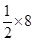= 4 sq. units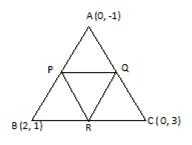P, Q and R are the mid–points of sides AB, AC and BC respectively.

Applying Section Formula to find the vertices of P, Q and R, we get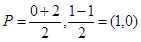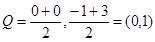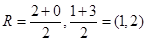Applying same formula, Area of △PQR =[1 (1 − 2) + 0 (2 − 0) + 1 (0 − 1)] =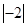= 1 sq. units (numerically)

Now,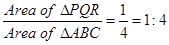NCERT Solutions for Class 10 Maths Exercise 7.3

###### 4. Find the area of the quadrilateral whose vertices taken in order are (–4, –2), (–3, –5), (3, –2) and (2, 3).

= Area of Triangle ABD +

Area of Triangle BCD … (1)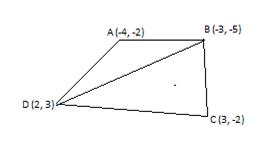Using formula to find area of triangle:

Area of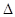ABD

==[−4 (−5 − 3) – 3 {3 − (−2)} + 2 {−2 − (−5)}]

=(32 – 15 + 6)

=(23) = 11.5 sq units … (2)

Again using formula to find area of triangle:

Area of △BCD ==[−3 (−2 − 3) + 3 {3 − (−5)} + 2 {−5 − (−2)}]

=(15 + 24 − 6)

=(33) = 16.5 sq units … (3)

Putting (2) and (3) in (1), we get

Area of Quadrilateral ABCD = 11.5 + 16.5 = 28 sq units

NCERT Solutions for Class 10 Maths Exercise 7.3

###### 5. We know that median of a triangle divides it into two triangles of equal areas. Verify this result for △ABC whose vertices are A (4, –6), B (3, –2) and C (5, 2).

Ans. We have △ABC whose vertices are given.

We need to show that ar(△ABD) = ar(△ACD).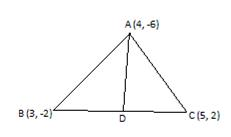Let coordinates of point D are (x, y)

Using section formula to find coordinates of D, we get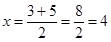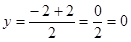Therefore, coordinates of point D are (4, 0)

Using formula to find area of triangle:

Area of △ABD ==[4 (−2 − 0) + 3 {0 − (−6)} + 4 {−6 − (−2)}]

=(−8 + 18 −16)

=(−6) = −3 sq units

Area cannot be in negative.

Therefore, we just consider its numerical value.

Therefore, area of △ABD = 3 sq units … (1)

Again using formula to find area of triangle:

Area of △ACD ==[4 (2 − 0) + 5 {0 − (−6)} + 4 {−6 −2 )}]

=(8 + 30 − 32) = ½ (6) = 3 sq units … (2)

From (1) and (2), we get ar(△ABD) = ar(△ACD)

Hence Proved.

## NCERT Solutions for Class 10 Maths Exercise 7.3

NCERT Solutions Class 10 Maths PDF (Download) Free from myCBSEguide app and myCBSEguide website. Ncert solution class 10 Maths includes text book solutions from Mathematics Book. NCERT Solutions for CBSE Class 10 Maths have total 15 chapters. 10 Maths NCERT Solutions in PDF for free Download on our website. Ncert Maths class 10 solutions PDF and Maths ncert class 10 PDF solutions with latest modifications and as per the latest CBSE syllabus are only available in myCBSEguide.

## CBSE app for Class 10

To download NCERT Solutions for Class 10 Maths, Computer Science, Home Science,Hindi ,English, Social Science do check myCBSEguide app or website. myCBSEguide provides sample papers with solution, test papers for chapter-wise practice, NCERT solutions, NCERT Exemplar solutions, quick revision notes for ready reference, CBSE guess papers and CBSE important question papers. Sample Paper all are made available through the best app for CBSE### 5 thoughts on “NCERT Solutions for Class 10 Maths Exercise 7.3”

1. good and clear solution

2. EXCELENT SOLUTION

3. Jo bhi to ap 3 vala glat kare ho area glat he

4. Nice solutions. Thank you for helping me.

5. Nice